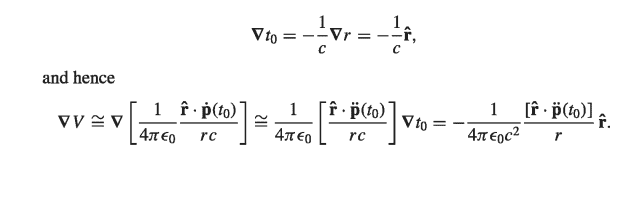# Potential vector of a oscilating dipole:

LCSphysicist
Homework Statement:
I.
Relevant Equations:
.
I am passing through some difficulties to understand the reasoning to derive the electric potential of an oscilating dipole used by Griffths at his Electrodynamics book:
Knowing that ##t_o = t - r/c##,What exactly he has used here to go from the first term after "and hence" to the second term? (The one involving ##\ddot p##). Maybe ##\nabla = \nabla t_o \frac{d}{dt_o}## ? But this does not make sense.

Homework Helper
Gold Member
A straightforward, but somewhat tedious, method is to work in cartesian coordinates.

Consider the ##x##-component of ##\nabla \left[ \frac {\mathbf{\hat r} \cdot \mathbf{ \dot p}(t_0)}{r} \right]##:

$$\left(\nabla \left[ \frac {\mathbf{\hat r} \cdot \mathbf{ \dot p}(t_0)}{r} \right]\right)_x = \frac{\partial}{\partial x} \left[ \frac{x}{r^2} \dot p_x(t_0) + \frac{y}{r^2} \dot p_y(t_0) +\frac{z}{r^2} \dot p_z(t_0) \right]$$
Expand this out. You can drop terms that fall off faster than ##1/r##.

When calculating ##\large \frac{\partial \dot p_x(t_0)}{\partial x}##, recall that ##t_0 = t-r/c =t-\sqrt{x^2+y^2+z^2}/c##. So, you can use the chain rule to write $$\frac{\partial \dot p_x(t_0)}{\partial x} = \frac{d \dot p_x}{dt_0} \frac{\partial t_0}{\partial x}$$
Likewise for dealing with ##\large \frac{\partial \dot p_y(t_0)}{\partial x}## and ##\large \frac{\partial \dot p_z(t_0)}{\partial x}##.

You should find that
$$\left(\nabla \left[ \frac {\mathbf{\hat r} \cdot \mathbf{ \dot p}(t_0)}{r} \right]\right)_x = \left( \frac {\mathbf{\hat{r}}}{r} \cdot \mathbf{\ddot p}(t_0)\right) \frac{\partial t_0}{\partial x}$$
Similar results are obtained for the ##y## and ##z## components of ##\nabla \left[ \frac {\mathbf{\hat r} \cdot \mathbf{ \dot p}(t_0)}{r} \right]##.

•Delta2
Staff Emeritus
$$[\nabla \dot{\vec p}(t_0)]_{ij} = \frac{\partial \dot p^j(t_0)}{\partial x^i} = \frac{d \dot p^j}{dt_0}\frac{\partial t_0}{\partial x^i} = \ddot{p}^j(t_0) \partial_i t_0.$$